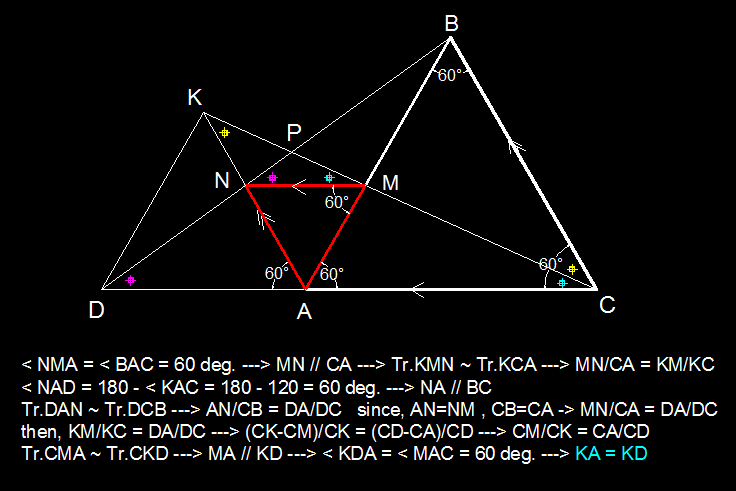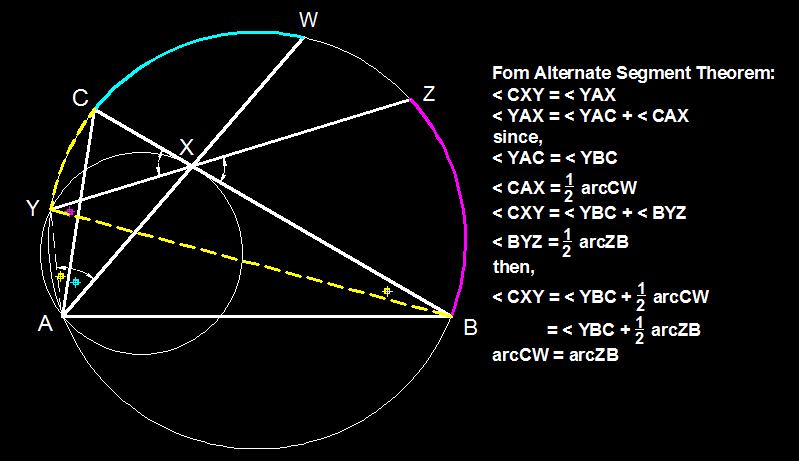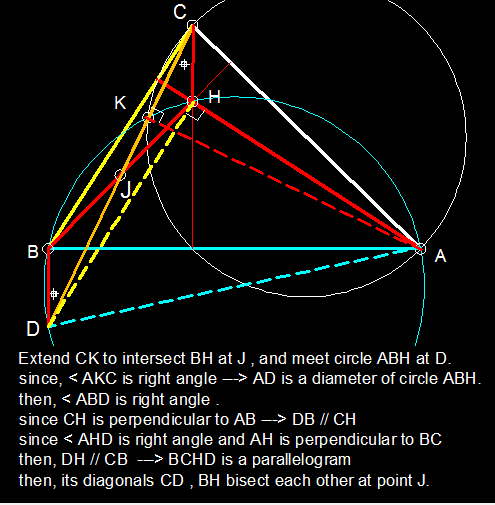# Australian School of Excellence 2015 Geometry Exam

• Each question is worth 7 points

• Time allowed is 4 hours

• No books, notes or calculators permitted

• Write full proofs with your answers

1) Let $\Gamma$ be the circumcircle of acute triangle $ABC$. Let $\omega$ be a circle passing through $A$ and tangent to $BC$ at $X$. Suppose that $\omega$ intersects $\Gamma$ for a second time at $Y$ where $Y$ lies on the minor arc $AC$ of $\Gamma$. The line $AX$ intersects $\Gamma$ for a second time at $W$. The line $XY$ intersects $\Gamma$ for a second time at $Z$.

Prove that the minor arcs $CW$ and $ZB$ of $\Gamma$ are equal in length.

2) Let $M$ be a point on side $AB$ of equilateral triangle $ABC$. The point $N$ is such that triangle $AMN$ is equilateral but $N$ does not lie on $AC$. Let $D$ be the intersection of lines $AC$ and $BN$. Let $K$ be the intersection of lines $CM$ and $AN$.

Prove $KA = KD$.

3) Let $X$, $Y$ and $Z$ be points on the sides $AD$, $AB$ and $AC$ respectively of rectangle $ABCD$.

Given that $AX = CZ$, prove that $XY + YZ \geq AC$.

4) Triangle $ABC$ satisfies $\angle ABC = 90^{\circ}$. Point $P$ lies on side $BC$, point $Q$ lies on side $AB$ and point $R$ lies inside triangle $ABC$ such that

$\angle PAB = \angle RAP = \angle CAR \quad \text{ and } \quad \angle BCQ = \angle QCR = \angle RCA.$

Prove that triangle $PQR$ is equilateral.

5) Let $H$ be the orthocentre of triangle $ABC$. The circle with diameter $AC$ intersects circle $ABH$ for a second time at point $K$.

Prove that line $CK$ bisects segment $BH$.Note by Sharky Kesa
5 years, 6 months ago

This discussion board is a place to discuss our Daily Challenges and the math and science related to those challenges. Explanations are more than just a solution — they should explain the steps and thinking strategies that you used to obtain the solution. Comments should further the discussion of math and science.

When posting on Brilliant:

• Use the emojis to react to an explanation, whether you're congratulating a job well done , or just really confused .
• Ask specific questions about the challenge or the steps in somebody's explanation. Well-posed questions can add a lot to the discussion, but posting "I don't understand!" doesn't help anyone.
• Try to contribute something new to the discussion, whether it is an extension, generalization or other idea related to the challenge.
• Stay on topic — we're all here to learn more about math and science, not to hear about your favorite get-rich-quick scheme or current world events.

MarkdownAppears as
*italics* or _italics_ italics
**bold** or __bold__ bold
- bulleted- list
• bulleted
• list
1. numbered2. list
1. numbered
2. list
Note: you must add a full line of space before and after lists for them to show up correctly
paragraph 1paragraph 2

paragraph 1

paragraph 2

[example link](https://brilliant.org)example link
> This is a quote
This is a quote
    # I indented these lines
# 4 spaces, and now they show
# up as a code block.

print "hello world"
# I indented these lines
# 4 spaces, and now they show
# up as a code block.

print "hello world"
MathAppears as
Remember to wrap math in $$ ... $$ or $ ... $ to ensure proper formatting.
2 \times 3 $2 \times 3$
2^{34} $2^{34}$
a_{i-1} $a_{i-1}$
\frac{2}{3} $\frac{2}{3}$
\sqrt{2} $\sqrt{2}$
\sum_{i=1}^3 $\sum_{i=1}^3$
\sin \theta $\sin \theta$
\boxed{123} $\boxed{123}$

## Comments

Sort by:

Top Newest

solution of problem 2 :- 4 years, 7 months ago

Log in to reply

Solution of problem 1 :- 4 years, 7 months ago

Log in to reply

solution of problem 5 :- 4 years, 7 months ago

Log in to reply

×

Problem Loading...

Note Loading...

Set Loading...